## Determine the number of roots the equation x^2+14x=-49 using the discriminant.

Question

Determine the number of roots the equation x^2+14x=-49 using the discriminant.

in progress 0
6 months 2021-07-15T02:52:45+00:00 2 Answers 13 views 0

Step-by-step explanation: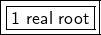Step-by-step explanation:

The quadratic formula is used to find the roots or zeroes of a quadratic equation. It is: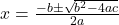The discriminant helps us find the number of roots. If the discriminant is…

• Negative: there are no real roots
• Zero: there is one real root
• Positive: there are two real roots

It is the expression under the square root symbol: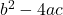First, we must put the given quadratic equation into standard form, which is: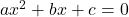The equation given is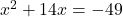. We have to move the -49 to the left side. Since it is a negative number, we add 49 to both sides.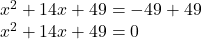Now we can solve for the discriminant because we know that:

• a= 1
• b= 14
• c= 49

Substitute these values into the formula for the discriminant.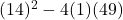Solve according to PEMDAS: Parentheses, Exponents, Multiplication, Division, Addition, Subtraction.

Solve the exponent.

• (14)²= 14 * 14= 196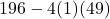Multiply 4, 1, and 49.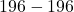Subtract.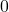The discriminant is zero, so the quadratic equation x²+ 14x = -49  has 1 real root.